Next: Optimization - General Bound Up: Conditional Densities: A Bayesian Previous: Conditional Densities: A Bayesian

## Conditional Density Estimation

Having obtained a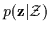or, more compactly a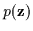, we can compute the probability of any point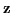in the vector space5.2. However, evaluating the pdf in such a manner is not necessarily the ultimate objective. Often, some components of the vector are given as input (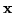) and the learning system is required the estimate the missing components as output5.3 (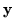). In other words,can be broken up into two sub-vectorsandand a conditional pdf is computed from the original joint pdf over the whole vector as in Equation 5.3. This conditional pdf is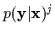with the j superscript to indicate that it is obtained from the previous estimate of the joint density. When an input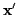is specified, this conditional density becomes a density over, the desired output of the system. This density is the required function of the learning system and if a final output estimate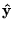is need, the expectation or arg max can be found via Equation 5.4.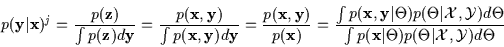(5.3)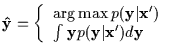(5.4)

Obtaining a conditional density from the unconditional (i.e. joint) probability density function in such a roundabout way can be shown to be suboptimal. However, it has remained popular and is convenient partly because of the availability of powerful techniques for joint density estimation (such as EM).

If we know a priori that we will need the conditional density, it is evident that it should be estimated directly from the training data. Direct Bayesian conditional density estimation is defined in Equation 5.5. The vector(the input or covariate) is always given and the(the output or response) is to be estimated. The training data is of course also explicitly split into the corresponding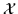and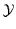vector sets. Note here that the conditional density is referred to as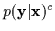to distinguish it from the expression in Equation 5.3.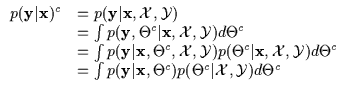(5.5)

Here,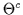parametrizes a conditional density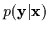.is exactly the parametrization of the conditional densitythat results from the joint density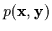parametrized by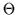. Initially, it seems intuitive that the above expression should yield exactly the same conditional density as before. It seems natural that p(y|x)c should equal p(y|x)j since theis just the conditioned version of. In other words, if the expression in Equation 5.1 is conditioned as in Equation 5.3, then the result in Equation 5.5 should be identical. This conjecture is wrong.

Upon closer examination, we note an important difference. Thewe are integrating over in Equation 5.5 is not the sameas in Equation 5.1. In the direct conditional density estimate (Equation 5.5), theonly parametrizes a conditional densityand therefore provides no information about the density ofor. In fact, we can assume that the conditional density parametrized byis just a function overwith some parameters. Therefore, we can essentially ignore any relationship it could have to some underlying joint density paramtrized by. Since this is only a conditional model, the term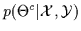in Equation 5.5 behaves differently than the similar term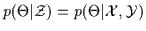in Equation 5.1. This is illustrated in the manipulation involving Bayes rule shown in Equation 5.6.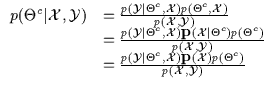(5.6)

In the final line of Equation 5.6, an important manipulation is noted: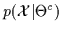is replaced with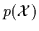. This implies that observingdoes not affect the probability of. This operation is invalid in the joint density estimation case sincehas parameters that determine a density in thedomain. However, in conditional density estimation, ifis not also observed,is independent from. It in no way constrains or provides information about the density ofsince it is merely a conditional density over. The graphical models in Figure 5.4 depict the difference between joint density models and conditional density models using a directed acyclic graph  . Note that themodel and theare independent ifis not observed in the conditional density estimation scenario. In graphical terms, thejoint parametrization is a parent of the children nodesand. Meanwhile, the conditional parametrizationand thedata are co-parents of the child(they are marginally independent). Equation 5.7 then finally illustrates directly estimated conditional density solution.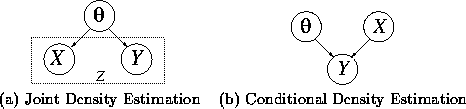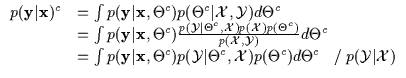(5.7)

The Bayesian integration estimate of the conditional density appears to be different and inferior from the conditional Bayesian integration estimate of the unconditional density. 5.4 The integral (typically) is difficult to evaluate. The corresponding conditional MAP and conditional ML solutions are given in Equation 5.8.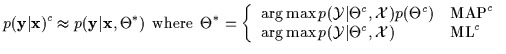(5.8)

At this point, the reader is encouraged to read the Appendix for an example of conditional Bayesian inference () and how it differs from conditioned joint Bayesian inference (). From this example we note that (regardless of the degree of sophistication of the inference) direct conditional density estimation is different and superior to conditioned joint density estimation. Since in many applications, full Bayesian integration is computationally too intensive, the MLc and the MAPc cases derived above will be emphasized. In the following, we shall specifically attend to the conditional maximum likelihood case (which can be extended to the MAPc) and see how General Bound Maximization (GBM) techniques can be applied to it. The GBM framework is a set of operations and approaches that can be used to optimize a wide variety of functions. Subsequently, the framework is applied to MLc and MAPc expressions that were advocated above to find their maximum. The result of this derivation is the Conditional Expectation Maximization (CEM) algorithm which will be the workhorse learning system we will be using for the ARL training data.Next: Optimization - General Bound Up: Conditional Densities: A Bayesian Previous: Conditional Densities: A Bayesian
Tony Jebara
1999-09-15